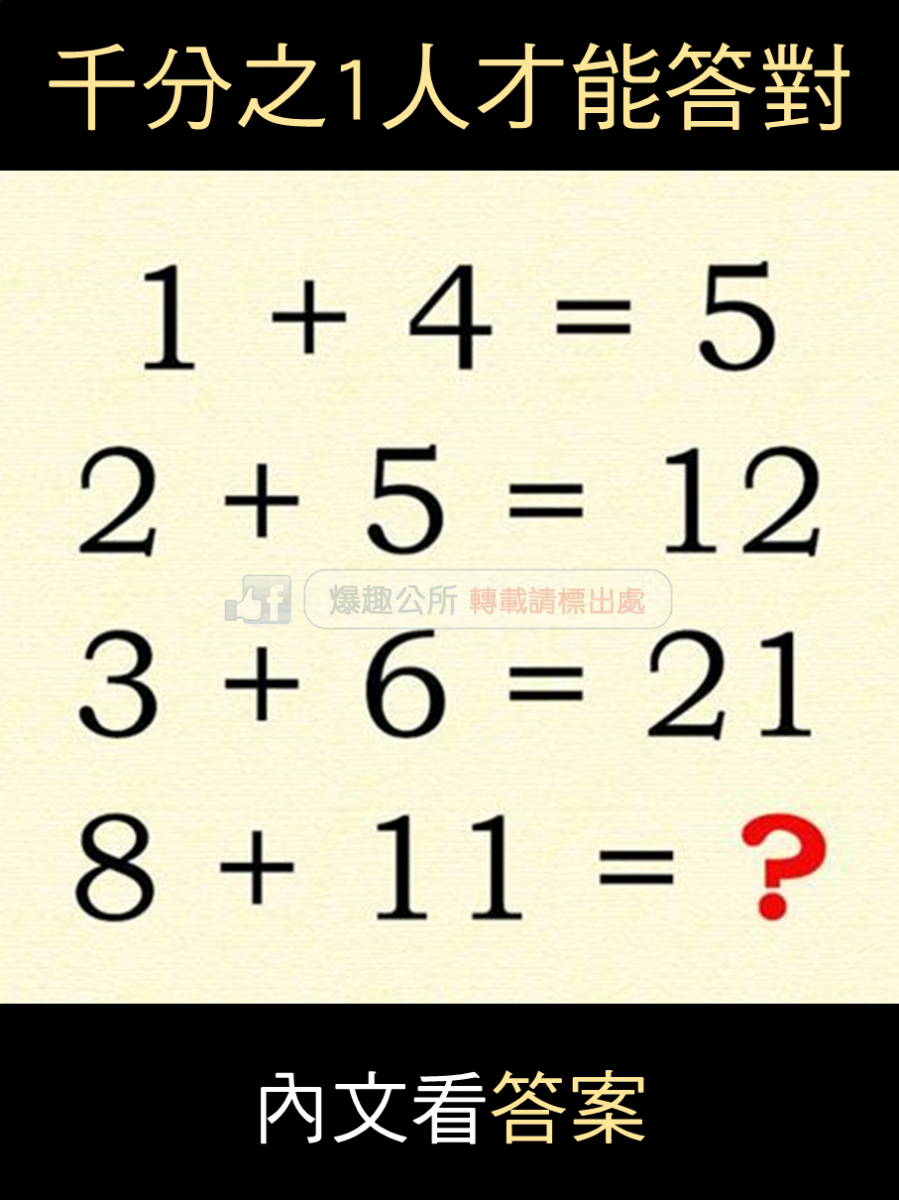1+4=5

（2+5）+5=12

（3+6）+12=21

（8+11）+21=40

1x（1+4）=5

2x（1+5）=12

3x（1+6）=21

8x（1+11）=96